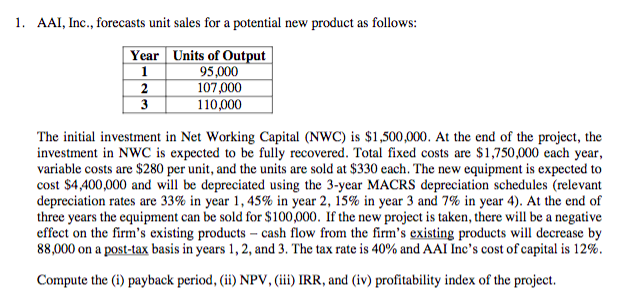1.AAI, Inc., forecasts unit sales for a potential new product as follows:Year Units of Output95,000107,000110,000The initial investment in Net Working Capital (NWC) is \$1,500,000. At the end of the project, theinvestment in NWC is expected to be fully recovered. Total fixed costs are \$1,750,000 each year,variable costs are \$280 per unit, and the units are sold at \$330 each. The new equipment is expected tocost S4,400,000 and will be depreciated using the 3-year MACRS depreciation schedules (relevantdepreciation rates are 33% in year 1, 45% in year 2, 15% in year 3 and 7% in year 4). At the end ofthree years the equipment can be sold for \$100,000. If the new project is taken, there will be a negativeeffect on the firm's existing products - cash flow from the firm's existing products will decrease by88,000 on a post-tax basis in years 1, 2, and 3. The tax rate s 40% and AAI Inc's cost ofcapital is 12%Compute the (i) payback period, (ii) NPV, (iii) IRR, and (iv) profitability index of the project.

Questionhelp_outlineImage Transcriptionclose1. AAI, Inc., forecasts unit sales for a potential new product as follows: Year Units of Output 95,000 107,000 110,000 The initial investment in Net Working Capital (NWC) is \$1,500,000. At the end of the project, the investment in NWC is expected to be fully recovered. Total fixed costs are \$1,750,000 each year, variable costs are \$280 per unit, and the units are sold at \$330 each. The new equipment is expected to cost S4,400,000 and will be depreciated using the 3-year MACRS depreciation schedules (relevant depreciation rates are 33% in year 1, 45% in year 2, 15% in year 3 and 7% in year 4). At the end of three years the equipment can be sold for \$100,000. If the new project is taken, there will be a negative effect on the firm's existing products - cash flow from the firm's existing products will decrease by 88,000 on a post-tax basis in years 1, 2, and 3. The tax rate s 40% and AAI Inc's cost ofcapital is 12% Compute the (i) payback period, (ii) NPV, (iii) IRR, and (iv) profitability index of the project. fullscreen
Step 1

Please see the table below. Since all the steps are sequential, I have converted the same into a tabular form.

Please be guided by the sign [+] / [-] against each variable in the first column.This will help you understand whether a particular variable has been added or subtracted.

Please be guided by the second column called "Linkage". This will help you understand the mathematics behind each row.

All the four subparts have been solved at appropriate place in the table itself.

Step 2
 Year, N Linkage 0 1 2 3 Purchase cost of new equipment A 4,400,000 Investment in working capital B 1,500,000 Total Initial Investment C = A + B 5,900,000 Depreciation schedule D 33% 45% 15% Depreciation E = D x A 1,452,000 1,980,000 660,000 Accumulated Depreciation F = Sum of E 1,452,000 3,432,000 4,092,000 Tax Basis (Asset balance) G = A - F 4,400,000 2,948,000 968,000 308,000 Units of Output H 95,000 107,000 110,000 Sale Price per unit I 330 330 330 Variable cost per unit J 280 280 280 Annual Operating Cash flows Revenue K = H x I 31,350,000 35,310,000 36,300,000 [-] Variable Costs L = H x J 26,600,000 29,960,000 30,800,000 [-] Fixed Costs M 1,750,000 1,750,000 1,750,000 [-] Depreciation E (as above) 1,452,000 1,980,000 660,000 EBIT N = K-L-M-E 1,548,000 1,620,000 3,090,000 [-] Taxes O = 40% x N 619,200 648,000 1,236,000 NOPAT P =N - O 928,800 972,000 1,854,000 [-] Post tax reduction in existing cash flows P' 88,000 88,000 88,000 Annual Operating Cash flows Q = P + E - P' 2,292,800 2,864,000 2,426,000 Sale value of the machine R 100,000 Gain / (Loss) on sale S = R - G (208,000) Tax on gain / loss T = S x 40% (83,200) Terminal Cash flows Post tax salvage value U = R - T 183,200 Release of working capital B (as above) 1,500,000 Terminal Cash flows V = U + B 1,683,200 Net Cash flows W = -C + Q + V (5,900,000) 2,292,800 2,864,000 4,109,200 Cumulative cash flows Sum of W (5,900,000) (3,607,200) (743,200) 3,366,000 Part (i) Payback Period 2.18 =2+743,200/4,109200 Cost of Capital X 12% Discount Factor Y = (1 + X)-N 1.0000 0.8929 0.7972 0.7118 PV of cash flow Z = W x Y (5,900,000) 2,047,143 2,283,163 2,924,847 Part (ii) NPV AA = Sum of Z 1,355,154 Part (iii) IRR 24% =IRR(-5900000,2292800,2864000,4109200) Part (iv) Profitability Index (AA + C) / C 1.23
...

Want to see the full answer?

See Solution

Want to see this answer and more?

Our solutions are written by experts, many with advanced degrees, and available 24/7

See Solution
Tagged in